## Domain and Range

### Learning Objectives

In this section, you will:

• Find the domain of a function defined by an equation.
• Graph piecewise-defined functions.

If you’re in the mood for a scary movie, you may want to check out one of the five most popular horror movies of all time—I am Legend, Hannibal, The Ring, The Grudge, and The Conjuring. (Figure) shows the amount, in dollars, each of those movies grossed when they were released as well as the ticket sales for horror movies in general by year. Notice that we can use the data to create a function of the amount each movie earned or the total ticket sales for all horror movies by year. In creating various functions using the data, we can identify different independent and dependent variables, and we can analyze the data and the functions to determine the domain and range. In this section, we will investigate methods for determining the domain and range of functions such as these.Figure 1. Based on data compiled by www.the-numbers.com.



### Finding the Domain of a Function Defined by an Equation

In Functions and Function Notation, we were introduced to the concepts of domain and range. In this section, we will practice determining domains and ranges for specific functions. Keep in mind that, in determining domains and ranges, we need to consider what is physically possible or meaningful in real-world examples, such as tickets sales and year in the horror movie example above. We also need to consider what is mathematically permitted. For example, we cannot include any input value that leads us to take an even root of a negative number if the domain and range consist of real numbers. Or in a function expressed as a formula, we cannot include any input value in the domain that would lead us to divide by 0.

We can visualize the domain as a “holding area” that contains “raw materials” for a “function machine” and the range as another “holding area” for the machine’s products. See (Figure).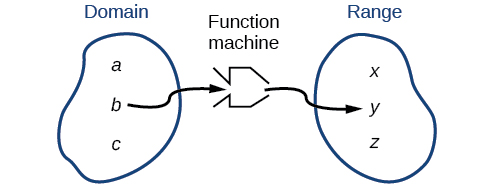Figure 2.

### Piecewise Function

A piecewise function is a function in which more than one formula is used to define the output. Each formula has its own domain, and the domain of the function is the union of all these smaller domains. We notate this idea like this:

$f\left(x\right)=\Bigg\{\begin{array}{l}\text{formula 1 if }x\text{ is in domain 1}\\ \text{formula 2 if }x\text{ is in domain 2}\\ \text{formula 3 if }x\text{ is in domain 3}\end{array}$

In piecewise notation, the absolute value function is

$|x|=\bigg\{\begin{array}{l}x\text{ if }x\ge 0\\ -x\text{ if }x<0\end{array}$

### How To

Given a piecewise function, write the formula and identify the domain for each interval.

1. Identify the intervals for which different rules apply.
2. Determine formulas that describe how to calculate an output from an input in each interval.
3. Use braces and if-statements to write the function.

### Writing a Piecewise Function

A museum charges $5 per person for a guided tour with a group of 1 to 9 people or a fixed$50 fee for a group of 10 or more people. Write a function relating the number of people,$\,n,\,$to the cost,$\,C.$

#### Analysis

The function is represented in (Figure). The graph is a diagonal line from$\,n=0\,$to$\,n=10\,$and a constant after that. In this example, the two formulas agree at the meeting point where$\,n=10,\,$but not all piecewise functions have this property.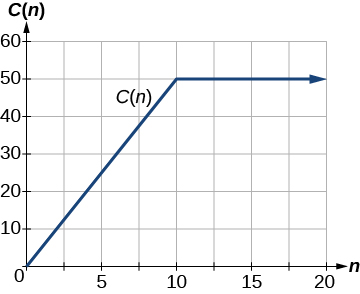Figure 23.

### Working with a Piecewise Function

A cell phone company uses the function below to determine the cost,$\,C,\,$in dollars for$\,g\,$gigabytes of data transfer.

$C\left(g\right)=\left\{\begin{array}{ccc}25& \text{if}& 0<g<2\\ 25+10\left(g-2\right)& \text{if}& g\ge 2\end{array}$

Find the cost of using 1.5 gigabytes of data and the cost of using 4 gigabytes of data.

#### Analysis

The function is represented in (Figure). We can see where the function changes from a constant to a shifted and stretched identity at$\,g=2.\,$We plot the graphs for the different formulas on a common set of axes, making sure each formula is applied on its proper domain.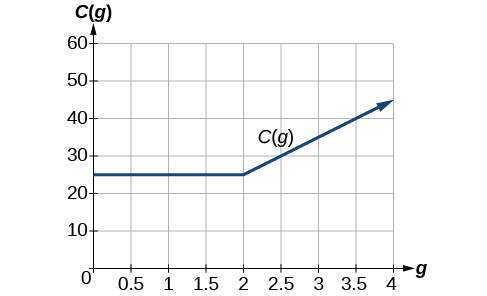Figure 24.

### How To

Given a piecewise function, sketch a graph.

1. Indicate on the x-axis the boundaries defined by the intervals on each piece of the domain.
2. For each piece of the domain, graph on that interval using the corresponding equation pertaining to that piece. Do not graph two functions over one interval because it would violate the criteria of a function.

### Graphing a Piecewise Function

Sketch a graph of the function.

$f\left(x\right)=\left\{\begin{array}{ccc}{x}^{2}& \text{if}& x\le 1\\ 3& \text{if}& 1<x\le 2\\ x& \text{if}& x>2\end{array}$

#### Analysis

Note that the graph does pass the vertical line test even at$\,x=1\,$and$\,x=2\,$because the points $\left(1,3\right)$ and $\left(2,2\right)$ are not part of the graph of the function, though $\left(1,1\right)$
and $\left(2,\,3\right)$ are.

### Try It

Graph the following piecewise function.

$f\left(x\right)=\left\{\begin{array}{ccc}{x}^{3}& \text{if}& x<-1\\ -2& \text{if}& -1<x<4\\ \sqrt{x}& \text{if}& x>4\end{array}$

Can more than one formula from a piecewise function be applied to a value in the domain?

No. Each value corresponds to one equation in a piecewise formula.

Access these online resources for additional instruction and practice with domain and range.

### Key Concepts

• The domain of a function includes all real input values that would not cause us to attempt an undefined mathematical operation, such as dividing by zero or taking the square root of a negative number.
• The domain of a function can be determined by listing the input values of a set of ordered pairs. See (Figure).
• The domain of a function can also be determined by identifying the input values of a function written as an equation. See (Figure), (Figure), and (Figure).
• Interval values represented on a number line can be described using inequality notation, set-builder notation, and interval notation. See (Figure).
• For many functions, the domain and range can be determined from a graph. See (Figure) and (Figure).
• An understanding of toolkit functions can be used to find the domain and range of related functions. See (Figure), (Figure), and (Figure).
• A piecewise function is described by more than one formula. See (Figure) and (Figure).
• A piecewise function can be graphed using each algebraic formula on its assigned subdomain. See (Figure).

### Section Exercises

#### Verbal

Why does the domain differ for different functions?

How do we determine the domain of a function defined by an equation?

Explain why the domain of$\,f\left(x\right)=\sqrt{x}\,$is different from the domain of$\,f\left(x\right)=\sqrt[]{x}.$

When describing sets of numbers using interval notation, when do you use a parenthesis and when do you use a bracket?

How do you graph a piecewise function?

#### Algebraic

For the following exercises, find the domain of each function using interval notation.

$f\left(x\right)=-2x\left(x-1\right)\left(x-2\right)$

$f\left(x\right)=5-2{x}^{2}$

$f\left(x\right)=3\sqrt{x-2}$

$f\left(x\right)=3-\sqrt{6-2x}$

$f\left(x\right)=\sqrt{4-3x}$

$\begin{array}{l}\\ f\left(x\right)=\sqrt[]{{x}^{2}+4}\end{array}$

$f\left(x\right)=\sqrt{1-2x}$

$f\left(x\right)=\sqrt{x-1}$

$f\left(x\right)=\frac{9}{x-6}$

$f\left(x\right)=\frac{3x+1}{4x+2}$

$f\left(x\right)=\frac{\sqrt{x+4}}{x-4}$

$f\left(x\right)=\frac{x-3}{{x}^{2}+9x-22}$

$f\left(x\right)=\frac{1}{{x}^{2}-x-6}$

$f\left(x\right)=\frac{2{x}^{3}-250}{{x}^{2}-2x-15}$

$\frac{5}{\sqrt{x-3}}$

$\frac{2x+1}{\sqrt{5-x}}$

$f\left(x\right)=\frac{\sqrt{x-4}}{\sqrt{x-6}}$

$f\left(x\right)=\frac{\sqrt{x-6}}{\sqrt{x-4}}$

$f\left(x\right)=\frac{x}{x}$

$f\left(x\right)=\frac{{x}^{2}-9x}{{x}^{2}-81}$

Find the domain of the function$\,f\left(x\right)=\sqrt{2{x}^{3}-50x}\,$by:

1. using algebra.
2. graphing the function in the radicand and determining intervals on the x-axis for which the radicand is nonnegative.

#### Graphical

For the following exercises, write the domain and range of each function using interval notation.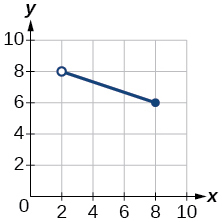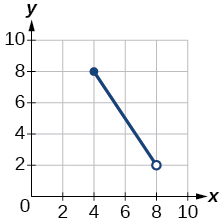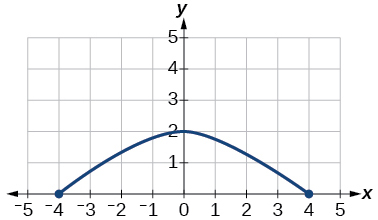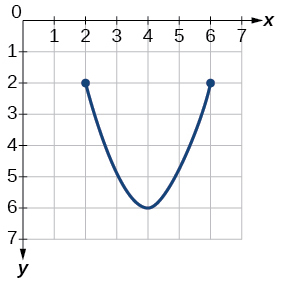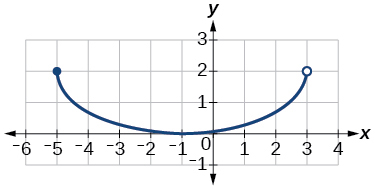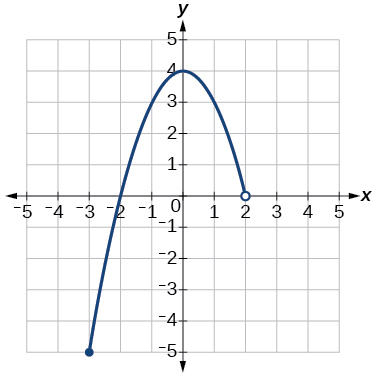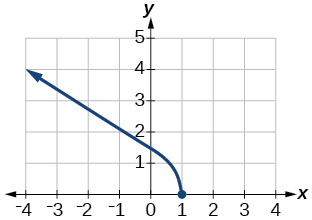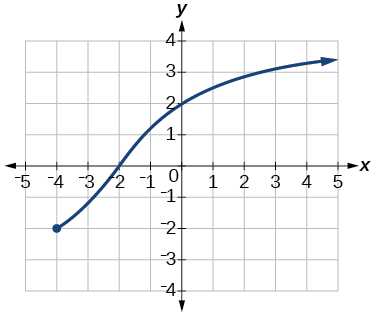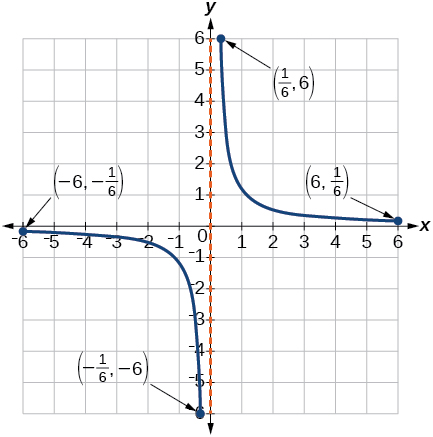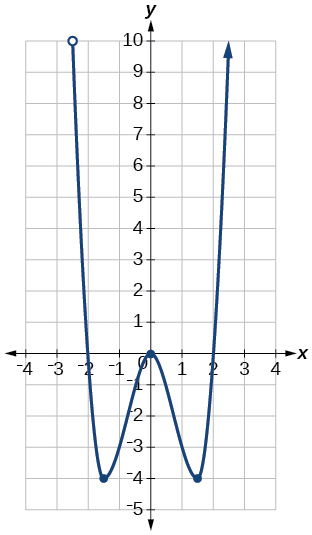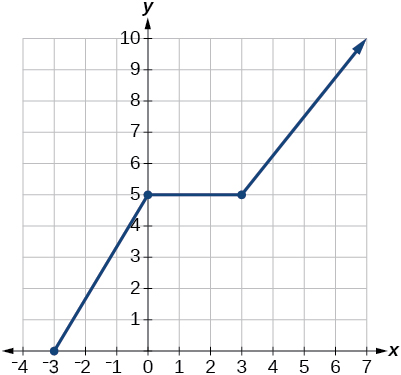For the following exercises, sketch a graph of the piecewise function. Write the domain in interval notation.

$f\left(x\right)=\left\{\begin{array}{lll}x+1\hfill & \text{if}\hfill & x<-2\hfill \\ -2x-3\hfill & \text{if}\hfill & x\ge -2\hfill \end{array}$

$f\left(x\right)=\left\{\begin{array}{lll}2x-1\hfill & \text{if}\hfill & x<1\hfill \\ 1+x\hfill & \text{if}\hfill & x\ge 1\hfill \end{array}$

$f\left(x\right)=\left\{\begin{array}{c}x+1\,\,\text{if}\,\,x<0\\ x-1\,\,\text{if}\,\,\,x>0\end{array}$

$f\left(x\right)=\left\{\begin{array}{ccc}3& \text{if}& x<0\\ \sqrt{x}& \text{if}& x\ge 0\end{array}$

$f\left(x\right)=\left\{\begin{array}{c}{x}^{2}\text{ if }x<0\\ 1-x\text{ if }x>0\end{array}$

$f\left(x\right)=\left\{\begin{array}{r}\hfill \begin{array}{r}\hfill {x}^{2}\\ \hfill x+2\end{array}\end{array}\,\,\begin{array}{l}\text{if}\,\,\,\,\,x<0\hfill \\ \text{if}\,\,\,\,\,x\ge 0\hfill \end{array}$

$f\left(x\right)=\left\{\begin{array}{ccc}x+1& \text{if}& x<1\\ {x}^{3}& \text{if}& x\ge 1\end{array}$

$f\left(x\right)=\left\{\begin{array}{c}|x|\\ 1\end{array}\begin{array}{l}\,\,\,\text{if}\,\,\,x<2\hfill \\ \,\,\,\text{if}\,\,\,x\ge 2\hfill \end{array}$

#### Numeric

For the following exercises, given each function $f,$evaluate $f\left(-3\right),\,f\left(-2\right),\,f\left(-1\right),$ and $f\left(0\right).$

$f\left(x\right)=\left\{\begin{array}{lll}x+1\hfill & \text{if}\hfill & x<-2\hfill \\ -2x-3\hfill & \text{if}\hfill & x\ge -2\hfill \end{array}$

$f\left(x\right)=\left\{\begin{array}{cc}1& \text{if }x\le -3\\ 0& \text{if }x>-3\end{array}$

$f\left(x\right)=\left\{\begin{array}{cc}-2{x}^{2}+3& \text{if }x\le -1\\ 5x-7& \text{if }x>-1\end{array}$

For the following exercises, given each function$\,f,\,$evaluate$f\left(-1\right),\,f\left(0\right),\,f\left(2\right),\,$and$\,f\left(4\right).$

$f\left(x\right)=\left\{\begin{array}{lll}7x+3\hfill & \text{if}\hfill & x<0\hfill \\ 7x+6\hfill & \text{if}\hfill & x\ge 0\hfill \end{array}$

$f\left(x\right)=\left\{\begin{array}{ccc}{x}^{2}-2& \text{if}& x<2\\ 4+|x-5|& \text{if}& x\ge 2\end{array}$

$f\left(x\right)=\left\{\begin{array}{ccc}5x& \text{if}& x<0\\ 3& \text{if}& 0\le x\le 3\\ {x}^{2}& \text{if}& x>3\end{array}$

For the following exercises, write the domain for the piecewise function in interval notation.

$f\left(x\right)=\left\{\begin{array}{c}x+1\,\,\,\,\,\text{ if}\,\,x<-2\\ -2x-3\,\,\text{if}\,\,x\ge -2\end{array}$

$f\left(x\right)=\left\{\begin{array}{c}{x}^{2}-2\,\,\,\,\,\text{ if}\,\,x<1\\ -{x}^{2}+2\,\,\text{if}\,\,x>1\end{array}$

$f\left(x\right)=\left\{\begin{array}{c}2x-3\\ -3{x}^{2}\end{array}\,\,\begin{array}{c}\text{if}\,\,\,x<0\\ \text{if}\,\,\,x\ge 2\end{array}$

#### Technology

Graph$\,y=\frac{1}{{x}^{2}}\,$on the viewing window$\,\left[-0.5,-0.1\right]\,$and$\,\left[0.1,0.5\right].\,$Determine the corresponding range for the viewing window. Show the graphs.

Graph$\,y=\frac{1}{x}\,$on the viewing window$\,\left[-0.5,-0.1\right]\,$and$\,\left[0.1,\text{ }0.5\right].\,$Determine the corresponding range for the viewing window. Show the graphs.

#### Extension

Suppose the range of a function$\,f\,$is$\,\left[-5,\text{ }8\right].\,$What is the range of$\,|f\left(x\right)|?$

Create a function in which the range is all nonnegative real numbers.

Create a function in which the domain is$\,x>2.$

#### Real-World Applications

The height$\,h\,$of a projectile is a function of the time$\,t\,$it is in the air. The height in feet for$\,t\,$seconds is given by the function$h\left(t\right)=-16{t}^{2}+96t.$
What is the domain of the function? What does the domain mean in the context of the problem?

The cost in dollars of making$\,x\,$items is given by the function$\,C\left(x\right)=10x+500.$

1. The fixed cost is determined when zero items are produced. Find the fixed cost for this item.
2. What is the cost of making 25 items?
3. Suppose the maximum cost allowed is \$1500. What are the domain and range of the cost function,$\,C\left(x\right)?$

### Glossary

interval notation
a method of describing a set that includes all numbers between a lower limit and an upper limit; the lower and upper values are listed between brackets or parentheses, a square bracket indicating inclusion in the set, and a parenthesis indicating exclusion
piecewise function
a function in which more than one formula is used to define the output
set-builder notation
a method of describing a set by a rule that all of its members obey; it takes the form$\,\left\{x|\,\text{statement about }x\right\}$

1. The Numbers: Where Data and the Movie Business Meet. “Box Office History for Horror Movies.” http://www.the-numbers.com/market/genre/Horror. Accessed 3/24/2014
2. http://www.eia.gov/dnav/pet/hist/LeafHandler.ashx?n=PET&s=MCRFPAK2&f=A.